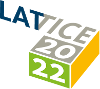#The 39th International Symposium on Lattice Field Theory (Lattice 2022)

Aug 8 – 13, 2022
Hörsaalzentrum Poppelsdorf
Europe/Berlin timezone

## Probing the R-ratio on the lattice

Aug 11, 2022, 10:00 AM
20m
CP1-HSZ/0.001 (CP1-HSZ) - HS3 (CP1-HSZ)

### CP1-HSZ/0.001 (CP1-HSZ) - HS3

#### CP1-HSZ

50
Show room on map
Oral Presentation QCD in searches for physics beyond the Standard Model

### Speaker

Alessandro De Santis (University and INFN of Rome Tor Vergata)

### Description

The ratio of the cross sections for $e^+e^- \to hadrons$ and $e^+e^-\to \mu^+\mu^-$ at c.o.m energy $E$, i.e. $R(E)$, is an extremely interesting observable. Its measurements are used in dispersive analyses of the leading hadronic vacuum polarization (HVP) contributing to the muon $g-2$, and the results of these analyses for a certain window observable are in significant tension with those coming from recent accurate lattice computations. It is thus very important to determine $R(E)$ from first-principles and compare it with experiment. In this talk we study $R(E)$ through a smearing in energy with different kernels $f(E)$. Indeed, by changing the shape of the smearing kernel one obtains an infinite number of observables, $R[f]$, that probe $R(E)$ in different ways. In particular, choosing $f(E) = \text{exp}(-Et)$ yields the Euclidean lattice correlator of two electromagnetic hadronic currents. This is a primary quantity from the lattice viewpoint, which we compare with its experimental counterpart directly obtained from the measured $R(E)$. We also use a recently proposed method for extracting smeared spectral densities from Euclidean lattice correlators in order to compute $R[f]$ for smearing kernels $f$ chosen as Gaussians of different width and central energy. Our still preliminary numerical results are obtained using state-of-the-art ETMC ensembles with $N=2+1+1$ dynamical quark flavours at three values of the lattice spacing ($\ge$ 0.06 fm), large volumes and physical pion mass.

### Primary authors

Alessandro De Santis (University and INFN of Rome Tor Vergata) Andrea Mosena (University of Rome Tor Vergata) Francesco Sanfilippo (INFN, Sezione Roma Tre) Giuseppe Gagliardi (INFN Sezione di Roma Tre) Nazario Tantalo (University and INFN of Rome Tor Vergata) Roberto Frezzotti (University and INFN of Roma Tor Vergata) Silvano Simula (INFN, Sezione Roma Tre) Simone Bacchio (The Cyprus Institute) Vittorio Lubicz (INFN, Sezione di Roma Tre,)